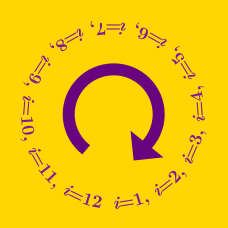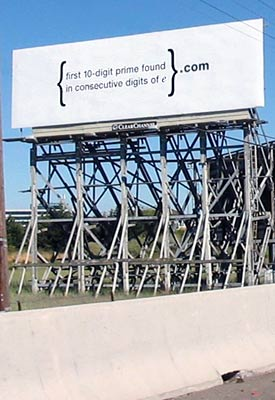Computer Science

# Loops: Level 2 Challenges

Your friend has written a program to check whether or not a number $n$ is prime. It is very simple and works by checking if $n$ is divisible by each number from 2 all the way to $n-1$. When you see this, you curse your friend and tell him he's wasting time.

If your friend didn't want to waste time, what is (approximately) the biggest number that he needs to check before he can determine that any number $n$ is prime?

\begin{aligned} 2^{2^0} +1&= & 3 \\ 2^{2^1} +1&= & 5 \\ 2^{2^2} +1&= & 17 \\ 2^{2^3} +1&= & 257 \\ 2^{2^4} +1&= & 65537 \\ \end{aligned}

Fact: The numbers above: $3,5,17,257,65537$ are all primes.

True or false?

"$2^{2^{5}} + 1$ is a prime."Find the first 10-digit prime number that is found in consecutive digits of $e$.

Background: The tech giant Google is known to have s​etup such billboards in the heart of Silicon Valley, and later in Cambridge, Massachusetts; Seattle, Washington; and Austin, Texas. It read

"{first 10-digit prime found in consecutive digits of e}.com".

Solving this problem and visiting the website led to an even more difficult problem to solve, which in turn led to Google Labs where the visitor was invited to submit a resume. Unfortunately, that website has been taken down.

Fibonacci sequence is defined as $F_0=0 , F_1=1$ and for $n \geq 2$ $F_n=F_{n-1}+F_{n-2}$

So the Fibonacci sequence is $0,1,1,2,3,5,8,13,...$

Find the sum of all the terms in the Fibonacci sequence which are less than $\textbf{1 billion}$ and are $\textbf{prime numbers}$.

Details and assumptions:-

$\bullet$ A prime number is the number which has only $2$ positive integer divisors, which are $1$ and the number itself. No other positive integer divides the number.

$\bullet$ 1 is not a prime number, 2 is the only even prime number.

This problem is a part of the set Crazy Fibonacci

How many 3-digit numbers satisfy:

$\overline { abc } =\quad { a }^{ 3 }+{ b }^{ 3 }+{ c }^{ 3 }$

×